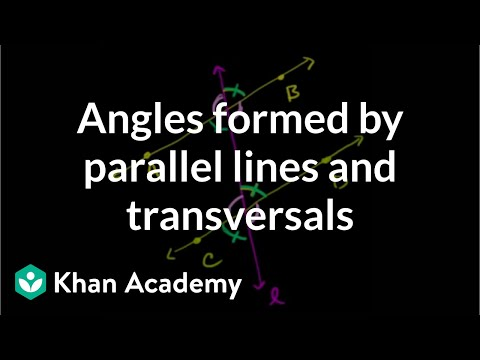Video

# Missing angles with a transversal (Full video)

Description: Learn how to find missing angle measurements in a figure with two parallel lines and a transversal. Created by Sal Khan. Let's say that we have two parallel lines. This is the symbol right over here to show that these two lines are parallel. Now, let's say that we know that this angle right over here is 110 degrees.

### Other videos you might be interested in### Angles in a triangle sum to 180° proof (Full video)